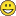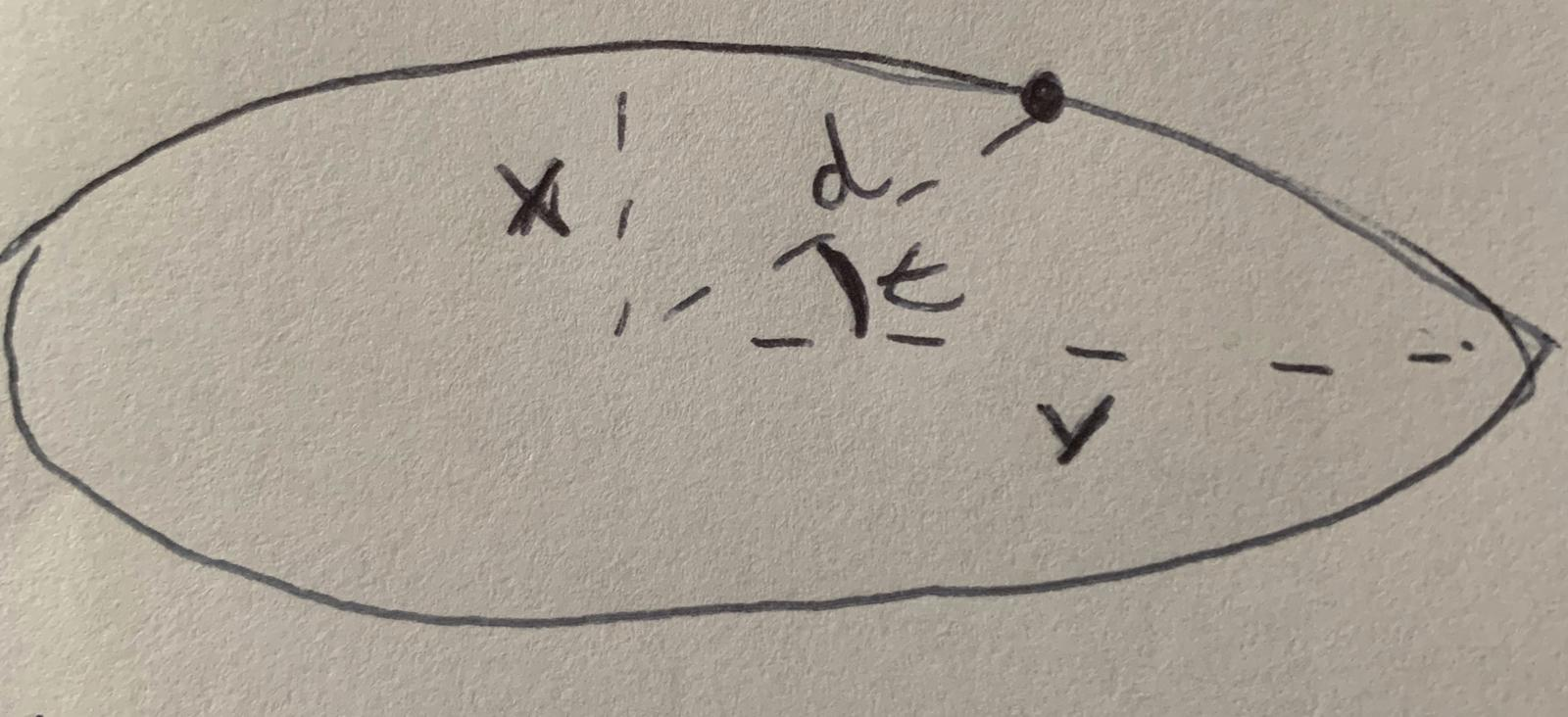# 4.14 - How to create an ellipse? (JS)

419
9
03-11-2020 12:56 PM
Highlighted

I want to transform the table below into graphics on the map as ellipses:I effectively want to create the 'Table to Ellipse' tool : Create Ellipse Tool ArcGIS 10 - YouTube

The only other thread I found was using the vertices taken from the draw tool:

UPDATE 1: I've created a codepen with one of the solutions given below, could someone provide assistance?

https://codepen.io/Jarviz/pen/yLNZqvQ?editors=1000

UPDATE 2: I've seen that some people recommended a geoprocessing server which then uses the 'Table to Ellipse' tool. Is this still a good idea?

Tags (2)
1 Solution

Accepted Solutions
Highlighted
byEsri Contributor

Hi Jack Jarvis‌,

Look like you are getting trouble with Math equation I gave as well.

Here is my codepen modified your codebase by adding getPointsForEllipse method to get ellipse points.

I just did quick ellipse only, so better check the distance of x-axis and y-axis based on what you need.9 Replies
Highlighted
byEsri Regular Contributor

Hi Jack, at this time we do not have plans to add support for ellipses in the 4x API. The thread you referenced is probably your best bet for now, unless someone else in the community has a better method.

Highlighted

Thank you for the response. Do you know of any sample data for ellipses that esri provide? Such as in a table format.

Highlighted
byEsri Contributor

Hi Jack Jarvis‌,

Since you know the center point, you just need to decide how far to go to east and north to draw the eclipse.

And find out a few point along the arc by using cartesian equation and create the clockwise ring from the north most point. And create the polygon by using these points into the ring.

Below is the formula you can reference.

I used that logic and draw pie polygon (part of circle).

Below is my code, you just play the formula at the method pointWithRadiumDistance.

``//getting another coordinate based on bearing and distance is started    degree2Radium: function (deg) {      return deg * (Math.PI / 180);    },    radium2Degree: function (rad) {      return rad * (180 / Math.PI);    },    pointWithRadiumDistance: function (lat1, lon1, bearing, distance) {      var rEarth = 6371.01; //# Earth's average radius in km      var epsilon = 0.000001; //# threshold for floating-point equality      var rlat1 = this.degree2Radium(lat1);      var rlon1 = this.degree2Radium(lon1);      var rbearing = this.degree2Radium(bearing);      //input is nautical mile, the formula is based on km,.. need to convert first, nm to km 1 => 1.852      var rdistance = (distance * 1.852) / rEarth; //# normalize linear distance to radian angle      //var rdistance = distance;      var rlat = Math.asin(        Math.sin(rlat1) * Math.cos(rdistance) +        Math.cos(rlat1) * Math.sin(rdistance) * Math.cos(rbearing)      );      var rlon = rlon1;      if (Math.cos(rlat) == 0 || Math.abs(Math.cos(rlat)) < epsilon) {        //# Endpoint a pole        rlon = rlon1;      } else {        rlon =          ((rlon1 -              Math.asin(                (Math.sin(rbearing) * Math.sin(rdistance)) / Math.cos(rlat)              ) +              Math.PI) %            (2 * Math.PI)) -          Math.PI;      }      var lat = this.radium2Degree(rlat);      var lon = this.radium2Degree(rlon);      return [lon, lat];    },    getPolygonByBearing: function (point, bearingArr, distance) {      var polygon = new Polygon(        new SpatialReference({          wkid: 4326        })      );      var polygonRing = [        [point.x, point.y]      ];      array.forEach(        bearingArr,        lang.hitch(this, function (bearDegree) {          var tmpPoint = this.pointWithRadiumDistance(            point.y,            point.x,            bearDegree,            distance          );          polygonRing.push(tmpPoint);        })      );      polygonRing.push([point.x, point.y]);      polygon.addRing(polygonRing);      return polygon;    }‍‍‍‍‍‍‍‍‍‍‍‍‍‍‍‍‍‍‍‍‍‍‍‍‍‍‍‍‍‍‍‍‍‍‍‍‍‍‍‍‍‍‍‍‍‍‍‍‍‍‍‍‍‍‍‍‍‍‍‍‍‍‍‍‍‍‍‍‍‍‍‍‍‍‍‍‍‍‍‍‍‍‍‍‍‍‍‍‍‍‍‍‍‍‍‍‍‍‍‍‍‍‍‍‍‍‍‍‍‍‍‍‍‍‍‍‍‍‍‍‍‍‍‍‍‍‍‍‍‍‍‍‍‍‍‍‍‍‍‍‍‍‍‍‍‍‍‍‍‍‍‍‍‍‍‍‍‍‍‍‍‍‍‍‍‍‍‍‍‍‍‍‍‍‍‍‍‍‍‍‍‍‍‍‍‍‍‍‍‍‍‍‍‍‍``
Highlighted
byEsri Contributor

Hi Jack Jarvis‌,

Quite interesting use case actually, let me know how's go.

Highlighted

I've reworded the original question to better explain what I'm trying to explain. I haven't had any luck with the provided solution from Than Aung.

Highlighted
byEsri Contributor

Hi Jack Jarvis‌,

I just give you the guideline how you can achieve. Anyway, I just take a look at your codepen.

There is one method usage which is require to fix.

The getPolygonByBearing method, second parameter should be array of angles.

One angle input will give you one point.

For your scenario=> eclipse, distance to travel shall be different based on the angle. (use the math at my earlier reply and find those)

After you collect a few points, you can feed points into your polygonEllipse variable.

`` const polygonEllipse = {          type: "polygon",          rings: getPolygonByBearing(          {            x: center_lat,            y: center_lon          },          [90, 80, 70, 60, 50, 40, 30, 20, 10, 0],          500,          ),        };‍‍‍‍‍‍‍‍‍‍‍‍‍‍‍‍‍‍‍‍‍‍``
Highlighted

Hi Than Aung,

Thank you that helps a lot, I think I'm slowly starting to grasp this. I'm trying to work out the last piece of the puzzle, the equation to get 'd' when I know the values for 'x', 'y' and 't'.Looking at the equation you reference:

Parametric Equation of an Ellipse - Math Open Reference

I don't see any reference to distance on the equation in order to find a point on the curve of the ellipse?

Highlighted
byEsri Contributor

Hi Jack Jarvis‌,

Look like you are getting trouble with Math equation I gave as well.

Here is my codepen modified your codebase by adding getPointsForEllipse method to get ellipse points.

I just did quick ellipse only, so better check the distance of x-axis and y-axis based on what you need.Highlighted

Hi Than Aung,

That makes a lot more sense to me now. A lot easier than I was expecting! Thank you a lot for all the time you put into helping.